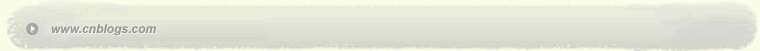C++分析研究 C++

 < 2013年6月 >
2627282930311
2345678
9101112131415
16171819202122
23242526272829
30123456

• 随笔 - 92
• 文章 - 4
• 评论 - 4
• 引用 - 0

# 文章档案

•

### 评论排行榜

这个题是个模拟，小数化分数的一般步骤可以看我的上一篇BLOG,无非除以10的位数次方，再约分一下，这个题也是如此。比较复杂的是无限小数的处理托福答案

分三种情况：

1、纯循环小数

2、纯不循环小数

3、混合小数

这三种的转化各有自己的性质，结论如下（证明过程可以见百度）

1、有限小数的话把小数点后面的数除以10（一位数）。100（两位数）。1000（三位数）等，

2、如果是无限循环小数那就把循环的数除以9、99、999（同上）

3、如果是混循环小数，循环数字为两位情况下不循环的数字一位则除以990,两位则9900,并加上不循环小数数值乘以990或者9900.

即：分子=不循环部分和循环部分连起来-不循环部分。分母=99（循环位数）0（不循环位数）

剩下的就是模拟字符串提取之类的细节问题了。另外要注意的是POW函数最好自己写，有的时候会丢失精度导致WA.

#include <iostream>

#include <string>

#include <cmath>

#include <stdlib.h>

using namespace std;

int gcd（int a,int b）

{

if（b==0）

return a;

return gcd（b,a%b）；

}

int lcm（int a,int b）

{

return （a*b）/gcd（a,b）；

}

int my_pow（int x,int n）

{

int res=1;

for（int i=1;i<=n;i++）

res*=x;

return res;

}

int main（）

{

int testcase;

cin》testcase;

for（int a=0;a<testcase;a++）

{

string tar;

string integerstr,loopstr;

int integer,loop;

int loopsize=0,intsize=0;

cin》tar;

if（tar.find（'（',0）==-1） //第一种情况，纯整数，不含循环节

{

for（int i=2;i<tar.size（）；i++）

{

integerstr+=tar[i];

intsize++;

}

integer=atoi（integerstr.c_str（））；

int cm;

cm=gcd（integer,my_pow（10,intsize））；

cout《integer/cm《"/"《my_pow（10,intsize）/cm《endl;

}

else if（tar=='（'） //第二种情况，纯循环小数

{

for（int i=3;i<tar.size（）-1;i++）

{

loopstr+=tar[i];

loopsize++;

}

loop=atoi（loopstr.c_str（））；

int div=my_pow（10,loopsize）-1;

int cm=gcd（loop,div）；

cout《loop/cm《"/"《div/cm《endl;

}

else if（tar.find（'（',0）！=-1||tar.find（'（',0）！=2 ）

{

int pos;

int intf;

int res;

for（int z=0;z<tar.size（）；z++）

{

if（tar[z]=='（'）

pos=z;

}

for（int i=2;i<pos;i++）

{

integerstr+=tar[i];

intsize++;

}

for（int j=pos+1;j<tar.size（）-1;j++）

{

loopstr+=tar[j];

loopsize++;

}

string fenzistr;

int fenzi;

fenzistr=integerstr+loopstr;

fenzi=atoi（fenzistr.c_str（））；

integer=atoi（integerstr.c_str（））；

loop=atoi（loopstr.c_str（））；

res=fenzi-integer;

string intfm; //例如0.32（56），原始分母为9900,0的个数等于非循环节的个数，9的个数等于循环节的个数

for（int p=0;p<loopsize;p++）

{

intfm+='9';

}

for（int o=0;o<intsize;o++）

{

intfm+='0';

}

intf=atoi（intfm.c_str（））；

int cm=gcd（res,intf）；

cout《res/cm《"/"《intf/cm《endl;

}

}

return 0;

}

posted on 2013-06-22 10:42 HAOSOLA 阅读(1171) 评论(0)  编辑 收藏 引用Copyright © HAOSOLA Powered by: 博客园 模板提供：沪江博客